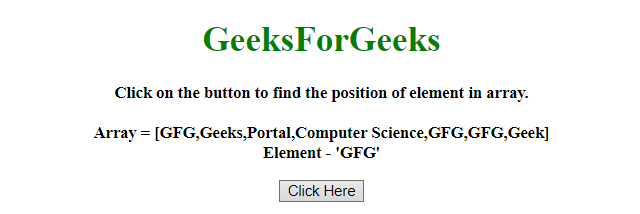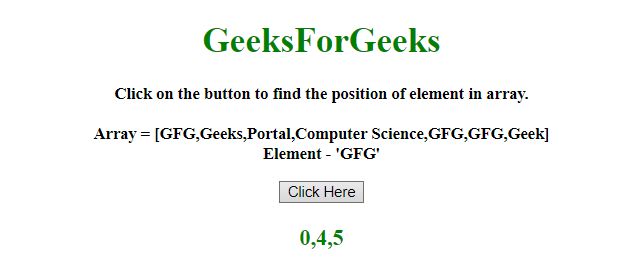# How to find the index of all occurrence of elements in an array using JavaScript ?

Given an array containing array elements and the task is to find all occurrence of an element from the array. We are going to do that with the help of JavaScript.

Approach 1: Declare an empty array which stores the indexes of all occurrence of the array element. Visit the array elements one by one using while loop and if the array elements match with the given element then push the index of element into an array. After visiting each element of the array, return the array of indexes.

Example: This example implements the above approach.

 `  ` `<``html``>  ` ` `  `<``head``>  ` `    ``<``title``>  ` `        ``How to find the index of all occurrences ` `        ``of element in an array using JavaScript? ` `    `` ` `  ` ` `  `<``body` `style` `= ``"text-align:center;"``>  ` `     `  `    ``<``h1` `style` `= ``"color:green;"` `>  ` `        ``GeeksForGeeks  ` `    `` ` `     `  `    ``<``p` `id` `= ``"GFG_UP"` `style` `= ` `        ``"font-size: 15px; font-weight: bold;"``> ` `    `` ` `     `  `    ``<``button` `onclick` `= ``"GFG_Fun()"``> ` `        ``Click Here ` `    `` ` `     `  `    ``<``p` `id` `= ``"GFG_DOWN"` `style` `= ` `        ``"color:green; font-size: 20px; font-weight: bold;"``> ` `    `` ` `     `  `    ``<``script``> ` `        ``var up = document.getElementById('GFG_UP');  ` `        ``var down = document.getElementById('GFG_DOWN');  ` `         `  `        ``var arr = [ ` `            ``'GFG', 'Geeks', 'Portal',  ` `            ``'Computer Science', 'GFG',  ` `            ``'GFG', 'Geek' ` `        ``]; ` `         `  `        ``var elm = 'GFG'; ` `         `  `        ``up.innerHTML = "Click on the button to find " ` `                    ``+ "the position of element in " ` `                    ``+ "array.<``br``><``br``>Array = ["  ` `                    ``+ arr + "]<``br``>" + "Element - '" ` `                    ``+ elm + "'"; ` `         `  `        ``function getInd(arr, val) { ` `            ``var index = [], i = -1; ` `            ``while ((i = arr.indexOf(val, i+1)) != -1){ ` `                ``index.push(i); ` `            ``} ` `            ``return index; ` `        ``} ` `     `  `        ``function GFG_Fun() { ` `            ``down.innerHTML = getInd(arr, elm); ` `        ``}  ` `    ``  ` `  ` ` `  ` `

Output:

• Before clicking on the button:• After clicking on the button:Approach 2: Visit the array elements one by one using reduce() method and if the array elements matches with the given element then push the index into the array. After visiting each element of the array, return the array of indexes.

Example: This example uses reduce() method to find the index of all occurrence of elements in an array.

 `  ` `<``html``>  ` ` `  `<``head``>  ` `    ``<``title``>  ` `        ``How to find the index of all occurrences ` `        ``of element in an array using JavaScript? ` `    `` ` `  ` ` `  `<``body` `style` `= ``"text-align:center;"``>  ` `     `  `    ``<``h1` `style` `= ``"color:green;"` `>  ` `        ``GeeksForGeeks  ` `    `` ` `     `  `    ``<``p` `id` `= ``"GFG_UP"` `style` `=  ` `        ``"font-size: 15px; font-weight: bold;"``> ` `    `` ` `     `  `    ``<``button` `onclick` `= ``"GFG_Fun()"``> ` `        ``Click Here ` `    `` ` `     `  `    ``<``p` `id` `= ``"GFG_DOWN"` `style` `=  ` `        ``"color:green; font-size: 20px; font-weight: bold;"``> ` `    `` ` `     `  `    ``<``script``> ` `        ``var up = document.getElementById('GFG_UP');  ` `        ``var down = document.getElementById('GFG_DOWN');  ` `         `  `        ``var arr = [ ` `            ``'GFG', 'Geeks', 'Portal', ` `            ``'Computer Science', 'GFG', ` `            ``'GFG', 'Geek' ` `        ``]; ` `         `  `        ``var elm = 'GFG'; ` `         `  `        ``up.innerHTML = "Click on the button to " ` `                ``+ "find the position of element" ` `                ``+ " in array.<``br``><``br``>Array = ["  ` `                ``+ arr + "]<``br``>" + "Element - '" ` `                ``+ elm + "'"; ` `         `  `        ``function GFG_Fun() { ` `            ``down.innerHTML =  ` `                ``arr.reduce(function(ind, el, i) { ` `                    ``if (el === 'GFG') ` `                        ``ind.push(i); ` `                    ``return ind; ` `                ``}, []);  ` `        ``}  ` `    ``  ` `  ` ` `  ` `

Output:

• Before clicking on the button:• After clicking on the button:My Personal Notes arrow_drop_upCheck out this Author's contributed articles.

If you like GeeksforGeeks and would like to contribute, you can also write an article using contribute.geeksforgeeks.org or mail your article to contribute@geeksforgeeks.org. See your article appearing on the GeeksforGeeks main page and help other Geeks.

Please Improve this article if you find anything incorrect by clicking on the "Improve Article" button below.

Improved By : nidhi_biet